# Peppers

In the box are yellow (a), green (b), and red (c) peppers. Their amount is in a ratio of 2:4:1. Most are yellow peppers and green the least. Calculate the number of peppers each type if the total number of peppers is 70.

a =  40
b =  10
c =  20

### Step-by-step explanation: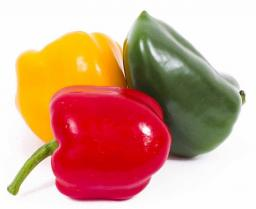Did you find an error or inaccuracy? Feel free to write us. Thank you!Tips to related online calculators
Check out our ratio calculator.

## Related math problems and questions:

• CandiesThere are red, blue and green candies in bad. Red to green is in 6:11 ratio and blue to red in a 7: 5 ratio. In what proportion are blue to green candies?
• Coloured numbersMussel wrote four different natural numbers with coloured markers: red, blue, green and yellow. When the red number divides by blue, it gets the green number as an incomplete proportion, and yellow represents the remainder after this division. When it div
• RatiosReduce the numbers: 50 in a 1:2 ratio 111 at a ratio of 2:3 70 at 10:50 560 at a ratio of 3:8
• Orange colour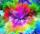In order for the painter to get orange, he has to mix yellow and red in a ratio of 3: 4. How many liters of orange did he mix if he used 8 liters of red?
• Two squaresTwo squares whose sides are in the ratio 5:2 have a sum of its perimeters 73 cm. Calculate the sum of the area of these two squares.
• Unknown number 5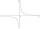Daniel thinks an integer. When he change this number at a ratio of 2:5, he got the number 2.8. Determine what number think Daniel.
• Moneys in triple ratioMilan, John and Lili have a total 344 euros. Their amounts are in the ratio 1:2:5. Determine how much each of them has?
• Holidays with grandmam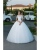We have packed three T-shirts - white, red, orange and five pairs of pants - blue, green, black, pink and yellow. How many days can we spend with the old mother if we put on a different combination of clothes every day?
• Money split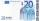Anton and Ferko got 2,500 euros together. The amount was split in a 1:4 ratio. How much did each get?
• Electric energyThere are 875 identical light bulbs in the sports hall lighting for 2 hours. How long does the same amount of electricity consume 100 such bulbs?
• The balls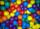You have 108 red and 180 green balls. You have to be grouped into the bags so that the ratio of red to green in each bag was the same. What smallest number of balls may be in one bag?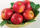Sadie picked up fallen apples from an apple tree in her yard. She picked up 14 green ones, 16 that were changing from green to red, and 18 that were red. What fraction in simplest form names the green apples?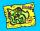What is the scale of the map, if 5.4 km is 72 mm on the map?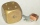Brass is an alloy of copper and zinc in a ratio of 3: 2. How many grams does a component that required 270 g of copper weigh?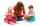The amount that Susan charges per hour for babysitting are directly proportional to the number of children she is watching. She charges \$4.50 for 3 kids. How much would she charge to babysit 5 kids?Divide the number 72 in the ratio 7: 2 and calculate the ratio of the numbers found in this order and write down as decimal.In order for the painter to get the desired color, he must mix green and yellow in a ratio of 4: 7. If it has 28 l of green color, how many liters of yellow color should he add? How many liters of mixed color does he get?